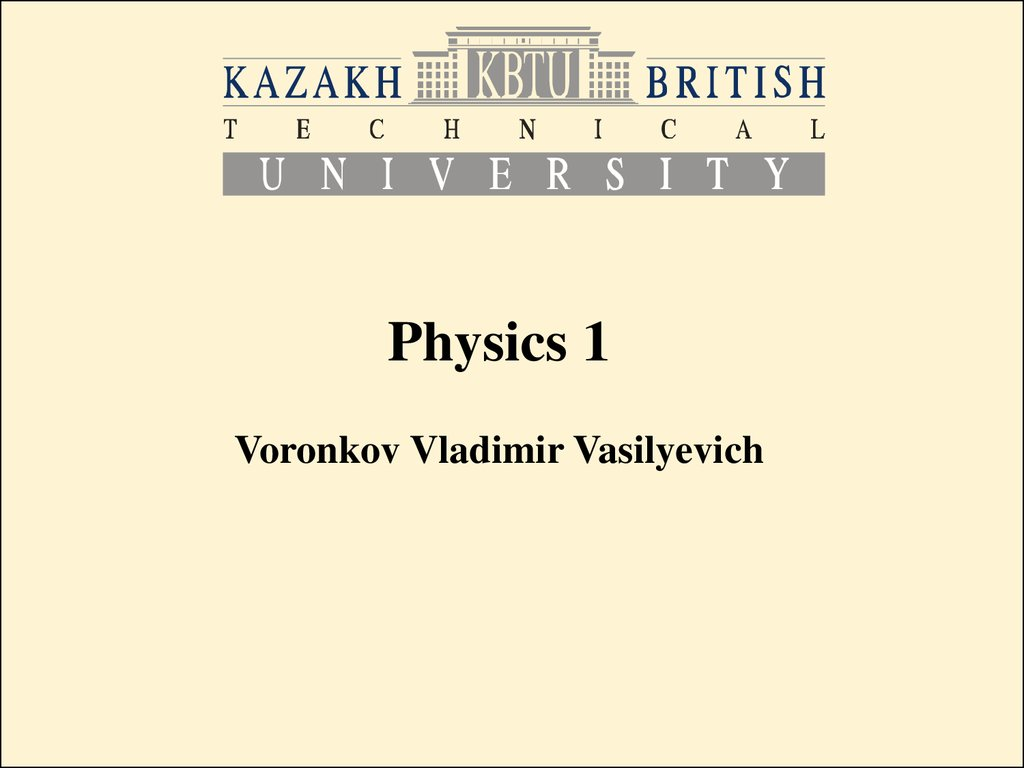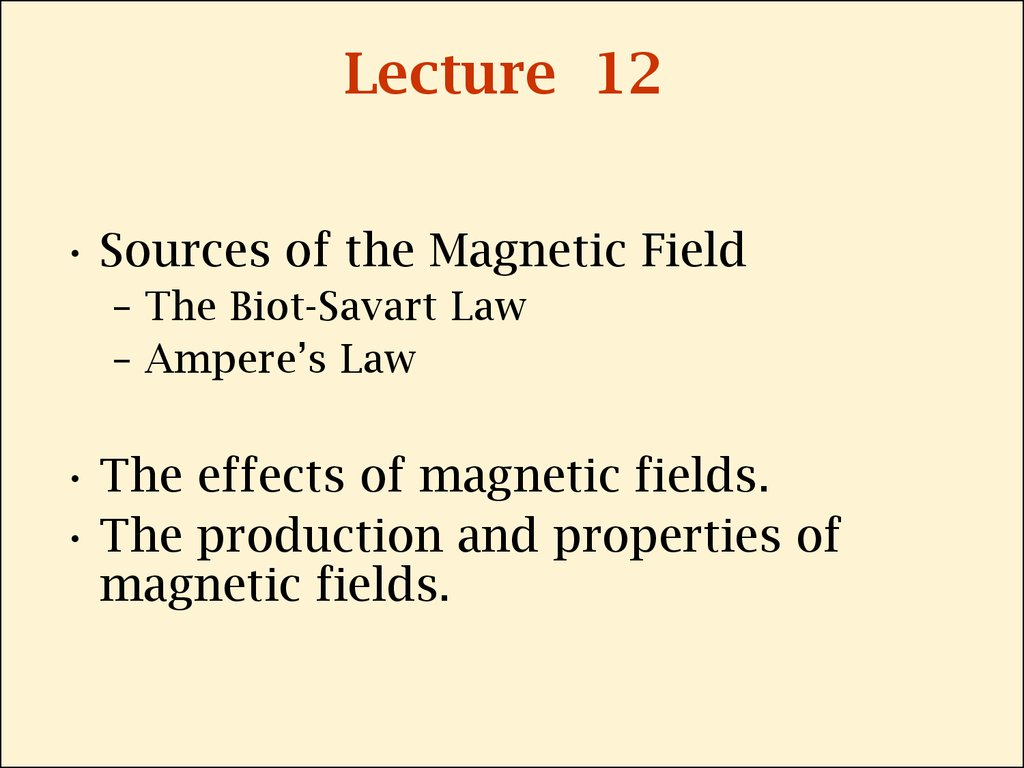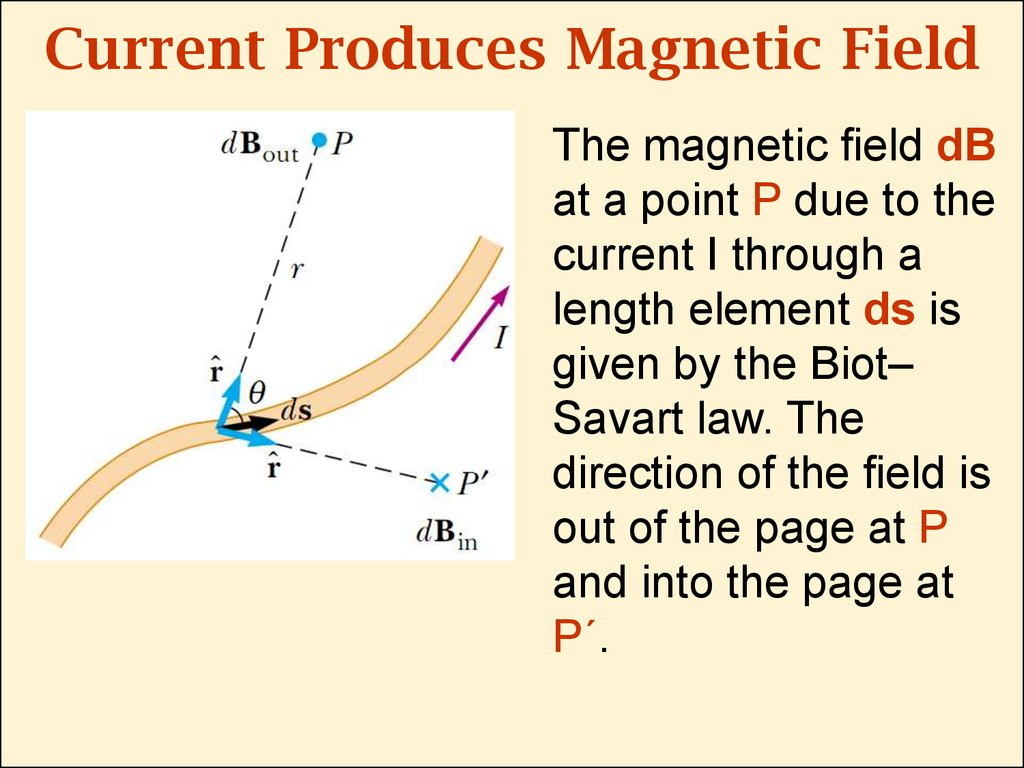# Sources of the мagnetic field/

Physics 1

## 2. Lecture 12

• Sources of the Magnetic Field
– The Biot-Savart Law
– Ampere’s Law
• The effects of magnetic fields.
• The production and properties of
magnetic fields.

## 3. Current Produces Magnetic Field

The magnetic eld dB
at a point P due to the
current I through a
length element ds is
given by the Biot–
Savart law. The
direction of the eld is
out of the page at P
and into the page at
P´.

## 4. The Biot-Savart Law

The experimental observations for the magnetic field
dB at a point P associated with a length element ds
of a wire carrying a steady current I:
•The vector dB is perpendicular both to ds (which
points in the direction of the current) and to the unit
vector rˆ directed from ds toward P.
•The magnitude of dB is inversely proportional to r2,
where r is the distance from ds to P.
•The magnitude of dB is proportional to the current and
to the magnitude ds of the length element ds.
•The magnitude of dB is proportional to sinQ, where Q
is the angle between the vectors ds and rˆ.

## 5.

• The foregoing experimental observations can be
expressed in one formula:
• Here dB is a magnetic force
at a point P associated with
a length element ds of a
current I.
• Unit vector
is directed from
ds toward P.
• r is the distance from ds to
P.
• m0 is the permeability of free
space:

## 6.

• Note that the eld dB in the Biot-Savart
law is the eld created by the current in
only a small length element ds of the
conductor. To nd the total magnetic eld
B created at some point by a current of
finite size, we must sum up contributions
from all current elements Ids that make
up the current. That is, we must
evaluate B by integrating over the entire
current distribution:

## 7. Magnetic Field of a Thin Straight Wire

• Using the Biot-Savart law
we can find the magnetic
field at point P, created by a
thin straight wire with current
in it:
• a is the distance from the
wire to P
Q1, Q2 are the angles shown
in the picture.

## 8. Magnetic Field of an Infinitely Long Wire

• For a very long thin straight wire we can
consider Q1=0, Q2=p, then:
• a is the distance from the wire to P
• I is the current in the wire
• This expression shows that the magnitude of
the magnetic field is proportional to the
current and decreases with increasing
distance from the wire.

## 9. Magnetic Field around a Wire

Because of the symmetry of the
wire, the magnetic field lines are
circles concentric with the wire
and lie in planes perpendicular to
the wire. The magnitude of B is
constant on any circle of radius a
and is given by the expression on
the previous slide:

## 10. Magnetic Force Between Two Parallel Conductors

Two long, straight, parallel
wires separated by distance
a and carrying currents I1
and I2 in the same direction.
The force exerted on one
wire due to the magnetic
eld set up by the other wire
is:
Parallel conductors carrying currents
- in the same direction attract each other.
- in opposite directions repel each other.

## 11. Ampere’s Law

• The line integral of B*ds around any closed
path equals m0I, where I is the total steady
current passing through any surface bounded
by the closed path.

## 12. Example for the Ampere’s Law

• We choose integration along
the path C:
• And finally we have the
result (cf. slide 7)

## 13. Magnetic Field of a Solenoid

• A solenoid is a long wire
wound in the form of a helix.
• Magnetic eld lines for a tightly
wound solenoid of nite length,
eld in the interior space is
strong and nearly uniform.

## 14.

Cross-sectional view of an
ideal solenoid, where the
interior magnetic eld is
uniform and the exterior
eld is close to zero.
Where
is number of turns per unit length.

## 15. Magnetic Flux

• The magnetic ux through an
area element dA is
B·dA = BdA cosQ
where dA is a vector
perpendicular to the surface and
has a magnitude equal to the
area dA.
• Therefore, the total magnetic
ux ФB through the surface is

## 16. Magnetic ﬂux through a plane lying in a magnetic ﬁeld

Magnetic ux through a plane lying in a magnetic eld
The ux through the plane is
zero when the magnetic eld
is parallel to the plane
surface.
The ux through the plane is
a maximum when the
magnetic eld is
perpendicular to the plane.

## 17. Gauss’s Law in Magnetism

• The net magnetic ux through any closed
surface is always zero:
• Here
is a scalar multiplication of two
vectors.
• Zero net magnetic flux through any closed
surface means that magnetic field lines has
no source. It is based on the fact that there
exist no magnetic monopoles.

## 18.

• The magnetic eld lines
of a bar magnet form
closed loops. Note that
the net magnetic ux
through a closed
surface surrounding
one of the poles (or any
other closed surface) is
zero. (The dashed line
represents the
intersection of the
surface with the page.)
• The number of lines
entering the surface
equals the number of
lines leaving it.

## 19. Displacement Current

• There is a charging capacitor,
with current I two imaginary
surfaces S1 and S2, and path P,
bounding to S1 and S2.
• When the path P is
considered as bounding S1,
then
because the conduction current
passes through S1.
• When the path is considered
as bounding S2, then
because no conduction current
passes through S2. Thus, we

## 20.

• This contradiction is resolved by introducing a
new quantity – the displacement current:
• Є0 is a free space permittivity, a constant
• ФЕ is the electric flux:
• As the capacitor is being charged (or
discharged), the changing electric eld
between the plates may be considered
equivalent to a current that acts as a
continuation of the conduction current in the
wire.

## 21. General form of Ampere’s Law

• So considering the displacement current, we
can write the General form of Ampere’s Law (or
Ampere-Maxwell law):

## 22.

• So the electric flux through
S2 is
• Where E is the electric field
between the plates, A is the
area of the plates, then
• So the electric flux through
S2 is

## 23.

• Then the displacement current through S2 is
• That is, the displacement current Id through
S2 is precisely equal to the conduction
current I through S1!

## 24. Units in Si

• Magnetic field
• Electric Field
• Magnetic permeability
of free space:
B T= N*s/(C*m)
T= N/(A*m)
E V/m=N/C